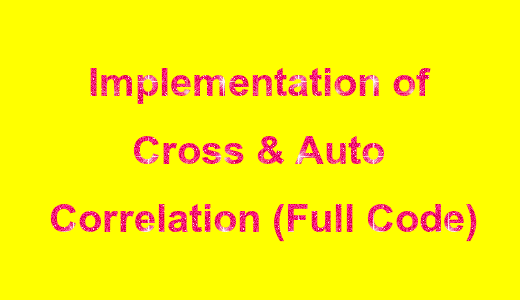# Implementation of Cross Correlation and Auto Correlation in Python: Full Source Code in PythonImplementation of Cross-Correlation and Auto Correlation in Python: Full Source Code in Python

What is the Correlation?
1. It defines the relationship which exists between one or more signals.
2. It shows the rate up to which the given signal resembles another signal.
What is Cross-Correlation?
When two different types of sequences are correlated, cross-correlation takes place.

What is Auto Correlation?
When the correlation between the two same sequences takes place, autocorrelation happens.

from convolution import linear_convolution

def correlation(x, ox, y= None, oy=None):

if y:

oy = len(y)-1

cross_corr = linear_convolution(x, y[:: -1], ox, oy)
print("The cross correlation of x and y is " , cross_corr, " with origin ", (ox+oy))

else:

ox_fold = len(x)-1 - ox

auto_corr = linear_convolution(x, x[:: -1], ox, ox_fold)
print("The auto correlation of x is " , auto_corr, " with origin " , (ox+ox_fold))

if __name__ == '__main__':

x   = [2, 3, 1, 4]

y   = [1, 3, 2, 1] ox, oy = 0, 0 correlation(x, ox) correlation(x, ox, y, oy)

Note: Please Insert Proper Indentations Wherever Required.